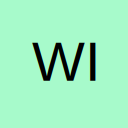Combined Loops for Userform Controls & Sheet Ranges

• Hi

I'm trying to simplify the code below and have previously been able to but am finding my aging brain repeatedly unable to fathom the answer.

I have a series of Checkboxes (numbered 73 to 99) that I need either a true or false value parsed to a respective cell within a range on a sheet.

Long hand looks like this:

Code
1. 'Loop Through Check Boxes
2. If CheckBox73.Value = True Then .Range("T8").Value = True Else .Range("T8").Value = False
3. If CheckBox74.Value = True Then .Range("T9").Value = True Else .Range("T9").Value = False
4. If CheckBox75.Value = True Then .Range("T10").Value = True Else .Range("T10").Value = False
5. If CheckBox76.Value = True Then .Range("T11").Value = True Else .Range("T11").Value = False
6. If CheckBox77.Value = True Then .Range("T12").Value = True Else .Range("T12").Value = False
7. If CheckBox78.Value = True Then .Range("T13").Value = True Else .Range("T13").Value = False
8.                 '---- etc etc
9. If CheckBox99.Value = True Then .Range("T34").Value = True Else .Range("T34").Value = False

But I know there is a method to use For Loops to loop through both the Checkboxes and the cell range [ .Range("T8:T34") ]

I tried various iterations of :

Any pointers of how far off of it working would be gratefully received.

Thanks all.

• The best way to do this will be to create a Class Module called clsCheckBox. In the class module you'll add the Click event code that ill run whenever a CheckBox is clicked.

Now you just need to attach the chkBox_Click event to each check box on your form.

Next to get the CheckBoxes to work with the correct range enter each range in the appropriate CheckBox's Tag Property as T8, etc.

See the example to see what I mean.